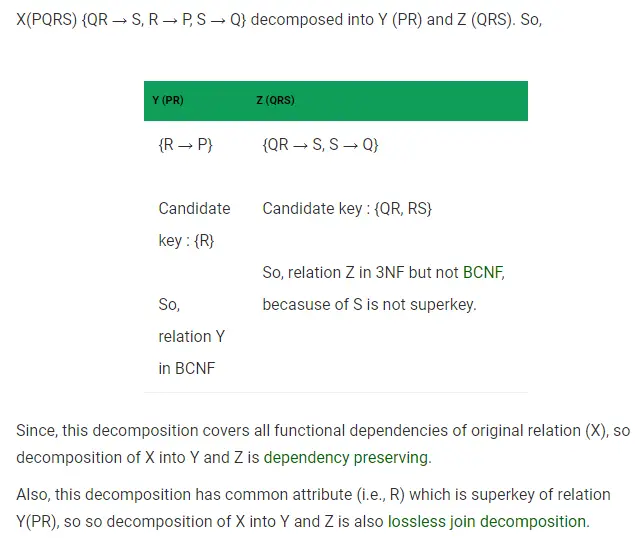# Gate CS-2019 Question Paper With Solutions

Q. 41 Let the set of functional dependencies F = {QR → S, R → P, S → Q} hold on a relation schema X = (PQRS). X is not in BCNF. Suppose X is decomposed into two schemas and Z where Y = (PR) and Z = (QRS). Consider the two statements given below:

• I. Both Y and Z are in BCNF
• II. Decomposition of X into Y and Z is dependency preserving and a lossless.

Which of the above statements is/are correct?

(A) I only

(B) Neither I nor II

(C) Both I and II

(D) II only